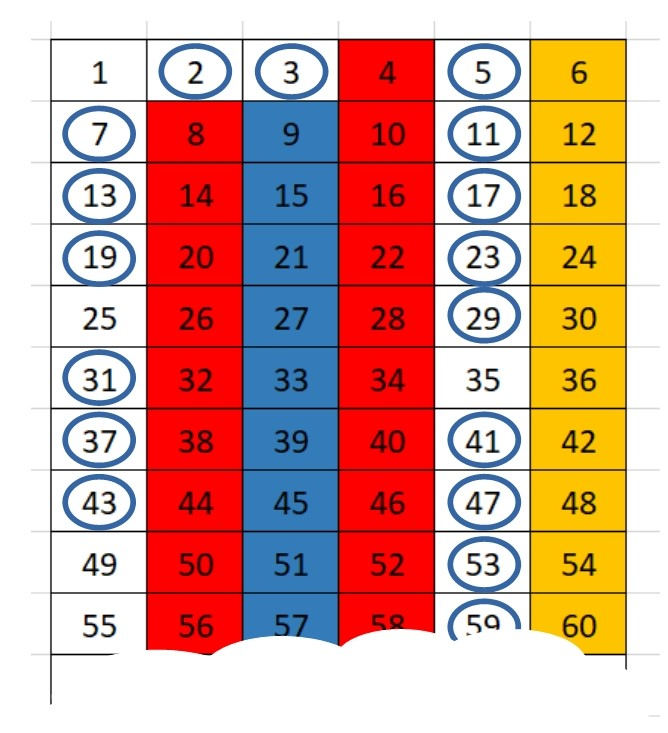#### You may also like### Latin Numbers

Can you create a Latin Square from multiples of a six digit number?### Summing Consecutive Numbers

15 = 7 + 8 and 10 = 1 + 2 + 3 + 4. Can you say which numbers can be expressed as the sum of two or more consecutive integers?### Number Sandwiches

Can you arrange the digits 1, 1, 2, 2, 3 and 3 to make a Number Sandwich?

# Where Are the Primes?

##### Age 11 to 16Challenge Level

Here are some prime numbers:
$5, 17, 23, 59, 89, 101$

They are all odd. What else do they have in common?

Add $1$ to each. What do the answers all have in common now?

Here are some more prime numbers:
$13, 19, 31, 37, 61, 67$

They are also all odd. What else do they have in common?

Subtract $1$ from each. What do the answers all have in common now?

Can you find any primes greater than $3$ which are not one more, or one less, than a multiple of $6$?

Charlie thought that it wasn't possible to find any primes which were not one more, or one less than a mutiple of $6$.  He thought that he might be able to use a number grid to help him prove this.Why do you think some numbers are shaded in red, blue or yellow?
What do you notice about the numbers in circles?

Claire also though that it wasn't possible to find any primes which were not one more, or one less than a mutiple of $6$.  She thought that she might be able to use some general expressions to help her prove this.

Any number can be written in the form $6n$, $6n+1$, $6n+2$, $6n+3$, $6n+4$ or $6n+5$.

We know that $6n$ is a multiple of $6$, so cannot be prime.
We can write $6n+2=2(3n+1)$, and so we know that $6n+2$ is a multiple of $2$.  The only multiple of $2$ which is prime is $2$ itself, which is not greater than $3$.

Can you complete Claire's method to show that primes numbers greater than $3$ must be of the form $6n+1$ or $6n+5$?

Can you show that you can write $6n+5$ in the form $6m-1$, and so show that numbers of the form $6n+5$ are one less than a multiple of $6$?

We are very grateful to the Heilbronn Institute for Mathematical Research for their generous support for the development of this resource.